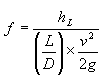Friction Factor Calculation

 Friction Factor (dimensionless)hL = head loss (feet of head) L = Length of tubing (feet) D = ID of tubing (feet) v = velocity (ft/sec) g = gravity (32.2 ft/s/s)